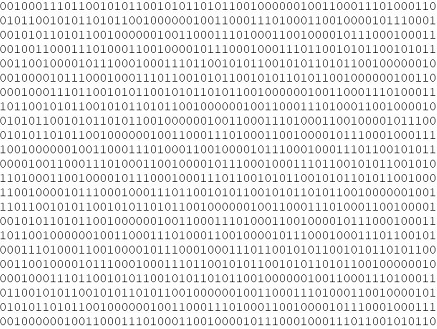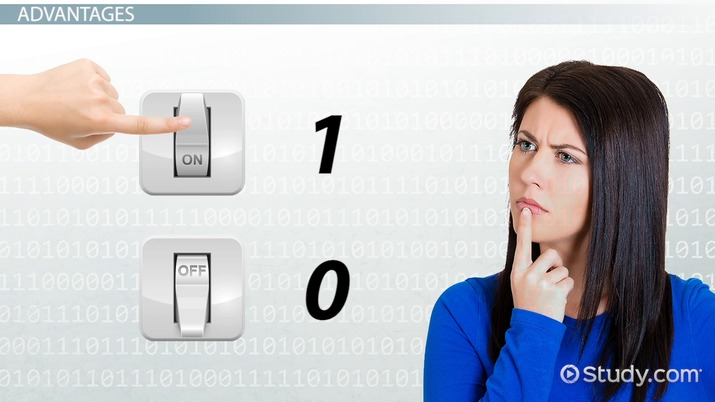# Binary Numbers & System

Dan Brioli, David Karsner, Kathryn Boddie
• Author
Dan Brioli

Dan has a B.A. in economics from the University of Pittsburgh and an A.A.S. in computer programming from the Pittsburgh Technical Institute. He has trained others in STEM topics and authored technical documentation throughout his career.

• Instructor
David Karsner

David holds a Master of Arts in Education

• Expert Contributor
Kathryn Boddie

Kathryn has taught high school or university mathematics for over 10 years. She has a Ph.D. in Applied Mathematics from the University of Wisconsin-Milwaukee, an M.S. in Mathematics from Florida State University, and a B.S. in Mathematics from the University of Wisconsin-Madison.

Find out what the binary system is. Study the binary numbers definition, examine a binary number example, and discover what the binary system is used for. Updated: 01/26/2022

Show

## Binary Numbers Definition

Every day, people across the world use a numeral system known as the base ten system. Another name for this numeral system is the decimal system, and in it, the digits 0-9 make up numbers that are used to perform all sorts of accounting and calculations.

But there are many other numeral systems out there in common use, including one being used by the computer or mobile phone that this text is being displayed on right now: the binary system.The binary system is also known as the base two numeral system. It uses only two digits, 0 and 1, but it can represent every number that the decimal system can. Other names for the binary system, depending on the industry it is being used in or its general application, are the on-off system, the go-no go system, or the open-closed system. Any system of measurement that records a pass/fail state or a yes/no state is essentially a binary system in its most basic form.

### History of Binary Numbers

The oldest recorded use of binary numeral systems were Horus-Eye fractions used as far back as 2400 BCE in the Fifth Dynasty of Egypt, with further evidence of their use in hieroglyphs from 1200 BCE. These were used for measuring fractions of liquid and grain.

Sometime in the 2nd century BCE, Indian scholars developed a binary system for describing the rhythms found within poetry. Short and long syllables were used to represent structure in a similar manner to Morse code.

The Enlightenment saw an increase in interest towards numeral systems and numbers, with researchers and mathematicians like Thomas Harriot and Francis Bacon experimenting with binary systems in some of their work during the 17th century. However, these were not very formalized works.

Gottfried Leibniz finally published a paper in the early 18th century titled "Explanation of Binary Arithmetic," detailing his findings on binary numbers after intense study of the Chinese I Ching. Later, George Boole would develop Boolean algebra, leading to a name commonly given to true/false variables and values in computer science (Boolean variables and types).An error occurred trying to load this video.

Try refreshing the page, or contact customer support.

Coming up next: What is the Binary Number System? - Definition & History

### You're on a roll. Keep up the good work!

Replay
Your next lesson will play in 10 seconds
• 0:03 What Is the Binary System?
• 0:56 Applications
• 2:57 Lesson Summary
Save Save

Want to watch this again later?

Timeline
Autoplay
Autoplay
Speed Speed

## Binary Numbers Examples

The binary number system is also known as the base two system due to each position in a numeral representing a power of 2. This is like how the decimal system (or base ten system) has positions that determine values measured in powers of 10. This is perhaps best illustrated with an example of several binary numerals and the math used to calculate their values:

2^6 = 64 2^5 = 32 2^4 = 16 2^3 = 8 2^2 = 4 2^1 = 2 2^0 = 1 Binary Numeral Decimal Numeral
0 1 0 0 1 1 1 100111 39
1 1 1 0 0 1 0 1110010 114
0 0 0 0 1 0 1 101 5

Note how each position is worth a certain value, just like in the decimal system, and when a 1 is present as a digit that value is added to the total of the binary value.

When the numeral system might be ambiguous, numbers are shown with their base as a subscript. Numbers in the decimal system will have a 10 as a subscript, while numbers in binary will have a 2 as their subscript:

{eq}\mathrm{Decimal}- 256_{10} {/eq}

{eq}\mathrm{Binary}- 256_{2} {/eq}

And like all other number systems, the binary system also has basic arithmetic operations that can be performed in it.

Addition in binary is like addition in decimal, except there are only two digits to work with. For example, adding the binary numbers 100111 and 1110:

    111  100111+001110_________=110101

In addition, 0 + 0 results in a 0, 1 + 0 results in a 1, and 1 + 1 results in a 0 and a carried 1 into the next digit's position. If a position results in a carried value being added to a calculation of 1 + 1, then the result for that position is 1 while also resulting in a carried 1. In the previous example, you can see this result in the 2^2 position.

### Subtraction

Subtraction is very similar to addition, of course, except carrying occurs based on what is being subtracted from the top number (just like in decimal). 0 - 0 results in a 0, 1 - 0 results in a 1, 1 - 1 results in 0, and 0 - 1 results in a 0 with the position to the left having 1 subtracted from it (since it is being borrowed to complete the calculation). If that would result in the position having a value less than zero, borrowing continues from the position further to the left. This again occurs at the 2^2 position in the example:

  100111 -001110_________=011001

### Multiplication

Multiplication is very simple in binary due to only having two digits. 0 times 0 results in 0, 0 times 1 results in 0, and 1 times 1 results in 1. The structure is similar to how multiplication is written in decimal:

    100111x           11__________    100111  100111=1110101

### Division

Division in binary is similar to long division in decimal, as well:

       001101      ________11|100111         11         ___           11           11           ___             101               11

To unlock this lesson you must be a Study.com Member.

• Activities
• FAQs

## Converting Numbers from Binary to Decimal

If you are given a number written in binary, you can translate it to a decimal by multiplying each binary bit by its power of two, and adding all the results. For example, the binary number 11101 converted to decimal is (1*(2^4)) + (1*(2^3)) + (1*(2^2)) + (0*(2^1)) + (1*(2^0)) = 16 + 8 + 4 + 0 + 1 = 29. In the following examples, convert the binary number to its equivalent decimal number.

• 1001
• 10101
• 11000

## Solutions

• For 1001 we have (1 * (2^3)) + (0 * (2^2)) + (0*(2^1)) + (1*(2^0)) = 8 + 1 = 9
• For 10101 we have (1 * (2^4)) + (0 * (2^3)) + (1 * (2^2)) + (0 * (2^1)) + (1 * (2^0)) = 16 + 4 + 1 = 21
• For 11000 we have (1 * (2^4)) + (1 * (2^3)) + (0 * (2^2)) + (0 * (2^1)) + (0 * (2^0)) = 16 + 8 = 24

## Converting Numbers from Decimal to Binary

We can also convert numbers written in decimal to binary numbers. To do this, first find the largest power of 2 that is less than or equal to the decimal number. Find the difference between the original number and this power of 2. Then find the largest power of 2 that is less than or equal to this new number. Repeat this process until you have no powers of 2 left. The decimal number is the sum of all the powers of 2 you found - to write the number in binary, put a 1 in for the bit representing each power of 2 you found, and a 0 in for all other bits. For example, for the decimal number 29, the highest power of 2 that is less than or equal to 29 is 2^4 = 16. The difference between 29 and 16 is 13. The highest power of 2 less than or equal to 13 is 2^3 = 8. The difference between 13 and 8 is 5. The highest power of 2 that is less than or equal to 5 is 2^2 = 4. The difference between 5 and 4 is 1. The highest power of 2 less than or equal to 1 is 2^0 = 1. This last difference is 0, so we are done. This means our decimal number of 29 can be written as (1*(2^4)) + (1*(2^3)) + (1*(2^2)) + (0*(2^1)) + (1*(2^0)) which makes the binary version of 29 the number 11101. In the following examples, convert the decimal number to binary.

• 19
• 33

## Solutions

• For 19, the highest power of 2 is 2^4 = 16. The difference is 3. The highest power of 2 for 3 is 2^1 = 2. The difference is 1. The last power of 2 needed is 2^0 = 1, and the last difference is 0. This means that the decimal number 19 can be written as (1*(2^4)) + (0*(2^3)) + (0*(2^2)) + (1*(2^1)) + (1*(2^0)) and so the binary version of 19 is 10011.
• For 33, the highest power of 2 is 2^5 = 32. The difference is 1. The highest power of 2 for 1 is 2^0 = 1. The difference is 0. This means that the decimal number 33 can be written as (1*(2^5)) + (0*(2^4)) + (0*(2^3)) + (0*(2^2)) + (0*(2^1)) + (1*(2^0)) thus the binary version of 33 is 100001.

#### What is an example of a binary system?

The binary numeral system is commonly used in computers because computers are composed of many electrical switches, which operate in two states: on and off. Due to the nature of electrical circuits operating in a binary way, binary numerals were an easy choice for how to represent numbers in low-level computer programs and hardware.

#### What is the binary system and how does it work?

The binary system is a numeral system designed to represent numbers using only 0 and 1 as its digits. Each "place" in a binary numeral or number stands for a power of 2, like how each "place" in the decimal system stands for a power of 10.

For example:

Binary 01001101 = Decimal 77

0 + 64 + 0 + 0 + 8 + 4 + 0 + 1 = 70 + 7

#### What is binary system in computer?

The binary system is used by low-level computer components to store and transmit data and to perform calculations based on on-off electrical signals or high-low voltage levels. It is also a system of digital representation of numbers and data stored by computer programs, and more generally a numeral system based around two digits (0 and 1).

### Register to view this lesson

Are you a student or a teacher?